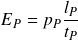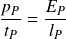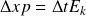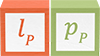# Momentum-energy symmetry

Momentum-energy symmetry is the conservation of Planck energy across the spatial and temporal distributions of an elementary particle. The symmetry is exemplified by the simple formula for kinetic energy in terms of a particle’s oscillation period

### rest mass

The formula shows that even the introduction of rest mass does not change the conservation of Planck energy across space and time.

For particles with no rest mass, the symmetry shows that Planck’s constant and the speed of light are conserved quantities. Changes in the particle’s wavelength are met with a proportional change in the particle’s oscillation period, and the particle’s velocity remains the speed of light. The symmetry is shown in the following illustration as a single vertical line across the wavelength and velocity unit dimensions.

For particles with rest mass, the symmetry requires that changes in wavelength are proportional to changes in velocity. The symmetry conserves Planck’s constant but is broken by rest mass which enables the particle to move at velocities less than light speed.

The symmetry is represented in the following illustration. The effect of rest mass is shown by a vertical line at the particle’s Compton wavelength—the limit of its velocity potential—the speed of light. The particle’s wavelength (the de Broglie wavelength) is shown as a vertical line across the spatial dimensions, and its velocity is shown by the broken lines across the 𝑑𝑥/𝑑𝑡 dimensions. When the change in position is the particle’s wavelength, the change in time is equal to the particle’s oscillation period.

## Derivation

At the Planck scale, energy is defined asThis second factor of 𝑙𝑃 / 𝑡𝑃 represents the dynamic property of motion that is often mistakenly attributed to momentum. At the maximum potential, a particle’s wavelength is equal to the Planck length, its mass and momentum are equal to the Planck mass and momentum, and its oscillation period is the Planck time.

The formula can be arranged to highlight relationships between the unit dimensions and to show how physical dynamics emerge from the equations. Restating the formula as an equalityproduces two forms of action in dimensions of momentum-length and energy-time, both in units of kgm2s-1. Each side of the equality conserves Planck’s constant.

The equation can also be stated asproducing two equivalent forms of force in dimensions of momentum per time and energy per length, both in units of kgms-2.

These two forms of the momentum-energy relationship are represented in the following illustration

Below the Planck scale potential, particles’ physical properties remain constrained by the momentum-energy symmetry according to the following relations:

### No rest mass### Rest massorJust as ƛ𝑚 and 𝑝𝑇𝜆 are conserved by the mass-momentum symmetry, the pairs of values ƛ𝑝 and 𝑇𝐸𝑘 are conserved by the momentum-energy symmetry. The former symmetry conserves the quantum constant 3.52 x 10-43 kgm and the latter conserves Planck’s constant, 1.05 x 10-34 kgm2s-1.

The momentum-energy symmetry can be summarized as follows:

ƛ𝑝 = 𝑇𝐸𝑘

### Potential### Invariant### Conserved quantity

1.05 x 10-34 kgm2s-1

1.05 x 10-34 kgm2s-1

The momentum-energy symmetry is demonstrated in the non-relativistic properties of particles with rest mass. The following table applies the rest mass, momentum, velocity, and spin operators on the maximum unit potentials of an electron. The operators are applied in stages for demonstration. Chosen states of the electron include the ground state, 2nd, and 3rd orbitals of an electron in the hydrogen atom. Applying the New Foundation Model operators gives results equal to those calculated with historical constants.

• * applies the ½ spin operator

Momentum-energy symmetry